References:

• T. Mitchell, 1997.
• P. Winston, 1992.

A decision tree is constructed by looking for regularities in data.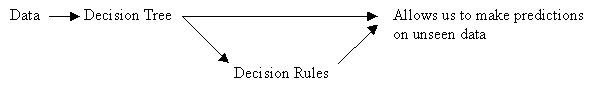From Data to Trees: Quinlan's ID3 Algorithm for Constructing a Decision Tree

General Form

Until each leaf node is populated by as homogeneous a sample set as possible:

• Select a leaf node with an inhomogeneous sample set.
• Replace that leaf node by a test node that divides the inhomogeneous sample set into minimally inhomogeneous subsets, according to an entropy calculation.

Specific Form

1. Examine the attributes to add at the next level of the tree using an entropy calculation.
2. Choose the attribute that minimizes the entropy.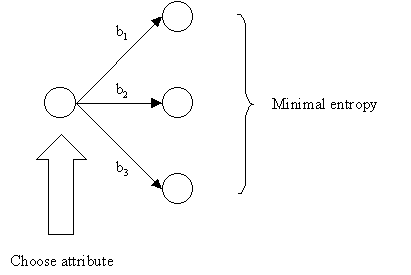Tests Should Minimize Entropy

It is computationally impractical to find the smallest possible decision tree, so we use a procedure that tends to build small trees.

Choosing an Attribute using the Entropy Formula

The central focus of the ID3 algorithm is selecting which attribute to test at each node in the tree.

Procedure:

1. See how the attribute distributes the instances.
2. Minimize the average entropy.
• Calculate the average entropy of each test attribute and choose the one with the lowest degree of entropy.

Review of Log2

On a calculator: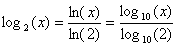eg) (0)log2(0) = -(the formula is no good for a probability of 0)
eg) (1)log2(1) = 0
eg) log2(2) = 1
eg) log2(4) = 2
eg) log2(1/2) = -1
eg) log2(1/4) = -2
eg) (1/2)log2(1/2) = (1/2)(-1) = -1/2

Entropy Formulae

Entropy, a measure from information theory, characterizes the (im)purity, or homogeneity, of an arbitrary collection of examples.

Given:

• nb, the number of instances in branch b.
• nbc, the number of instances in branch b of class c. Of course, nbc is less than or equal to nb
• nt, the total number of instances in all branches.

Probability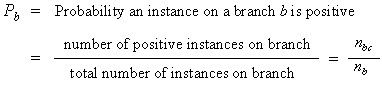• If all the instances on the branch are positive, then Pb = 1 (homogeneous positive)
• If all the instances on the branch are negative, then Pb = 0 (homogeneous negative)

Entropy

•• As you move from perfect balance and perfect homogeneity, entropy varies smoothly between zero and one.
• The entropy is zero when the set is perfectly homogeneous.
• The entropy is one when the set is perfectly inhomogeneous.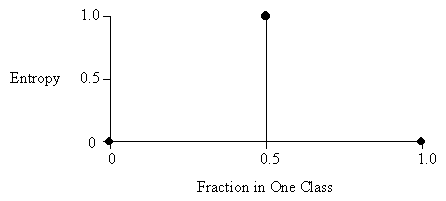The disorder in a set containing members of two classes A and B, as a function of the fraction of the set beloning to class A.
In the diagram above, the total number of instances in both classes combined is two.
In the diagram below, the total number of instances in both classes is eight.Average Entropy

•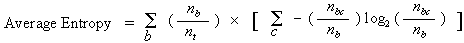Unlike the version space candidate-elimination algorithm,

• ID3 searches a completely expressive hypothesis space (ie. one capable of expressing any finite discrete-valued function), and thus avoids the difficulties associated with restricted hypothesis spaces.
• ID3 searches incompletely through this space, from simple to complex hypotheses, until its termination condition is met (eg. until it finds a hypothesis consistent with the data).
• ID3's inductive bias is based on the ordering of hypotheses by its search strategy (ie. follows from its search strategy).
• ID3's hypothesis space introduces no additional bias.

Approximate inductive bias of ID3: shorter trees are preferred over larger trees.

A closer approximation to the inductive bias of ID3: shorter trees are preferred over longer trees. Trees that place high information gain attributes close to the root are preferred over those that do not.

By viewing ID3 in terms of its search space and search strategy, we can get some insight into its capabilities and limitations:

• ID3's hypothesis space of all decision spaces is a complete space of finite discrete-valued functions, relative to the available attributes.
• Because every finite discrete-valued function can be represented by some decision tree, ID3 avoids one of the major risks of methods that search incomplete hypothesis spaces (such as version space methods that consider only conjunctive hypotheses): that the hypothesis space might not contain the target function.
• ID3 maintains only a single current hypothesis as it searches through the space of decision trees.
• This contrasts, for example, with the earlier version space candidate-elimination method, which maintains the set of all hypotheses consistent with the available training examples.
• However, by determining only a single hypothesis, ID3 loses the capabilities that follow from explicitly representing all consistent hypotheses.
• For example, it does not have the ability to determine how many alternative decision trees are consistent with the available training data, or to pose new instance queries that optimally resolve among these competing hypotheses.
• ID3, in its pure form, performs no backtracking in its search (greedy algorithm).
• Once it selects an attribute to test at a particular level in the tree, it never backtracks to reconsider this choice; it is susceptible to the usual risks of hill-climbing search without backtracking: converging to locally optimal solutions that are not globally optimal.
• In the case of ID3, a locally optimal solution corresponds to the decision tree it selects along the single search path it explores. However, this locally optimal solution may be less desirable than trees that would have been encountered along a different branch of the search.

(Although the pure ID3 algorithm does not backtrack, we discuss an extension that adds a form of backtracking: post-pruning the decision tree. See decision rules.)

• ID3 uses all training examples at each step in the search to make statistically based decisions regarding how to refine its current hypothesis.
• This contrasts with methods that make decisions incrementally, based on individual training examples (eg. version space candidate-elimination).
• One advantage of using statistical properties of all the examples is that the resulting search is much less sensitive to errors in individual training examples. ID3 can be easily extended to handle noisy training data by modifying its termination criterion to accept hypotheses that imperfectly fit the training data.